The VBA Range Object represents a cell or multiple cells in your Excel worksheet. Properties and Methods of the Range Object are used to manipulate cell values, change formatting and return attributes.

A Property stores information about the object, such as its address (\$A\$1, for example), its column number, and even its font name. These object properties can be assigned to other variables or can be set to new values.

A Method, on the other hand, is an action the object can perform. The Range Object can perform many actions, including being selected, cleared, copied and sorted.

In this tutorial, I’ll introduce you to a few Range Object Properties and Methods. Of course, Chapter 2 is titled “Getting Around,” so it’s only fitting that I focus on navigating your spreadsheet with the Range Object.

#### Range Arguments

The VBA Range Object accepts two arguments: Cell1 and Cell2. The second argument is optional, as denoted by the brackets, [ ], in the following image: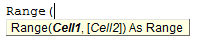You can pass an address to the Range Object with a string or with the Cells property. Standard address string notation is quite similar to how you would reference a cell in Excel. “B3” or “\$B\$3” would denote the third row, second column. “A1:B3” or “\$A\$1:\$B\$3” would represent the entire range from the first row, first column to the third row, second column.

The easiest way to learn is by playing around, so let’s start playing around. Open up the Visual Basic Editor and create a macro titled RangeDemo(). You’ll see the following pairs are equivalent:

``````Sub RangeDemo()
Range("A1")=7
Range(Cells(1, 1), Cells(1, 1))=7
End Sub``````

As are:

``````Sub RangeDemo()
Range("A1:C4") = 9
Range(Cells(1, 1), Cells(4, 3)) = 9
End Sub``````

#### VBA Range.Cells Property

You’ll notice the second item in each of the previous examples references an argument called Cells. The Cells property, or Range.Cells property, accepts two arguments: Row first, Column second.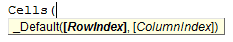Each argument must be an integer (a whole number). Specifically, each argument must be an integer greater than 0 since Excel row numbers and column numbers start from 1.

If you’re using the Cells Property to define your range, you’ll need to enter both arguments in the Range Object (Cell1 and Cell2). If you’re using a string, you really only need one.

Although defining your range with the Cells Property is longer, you’ll find it useful when you start looping through ranges in Excel (More on this in a future chapter).

#### Named Ranges

Let’s say you have a range named DisneyParks. You would reference the named range just like you would use string address notation.

``````Sub RangeDemo()
Range("DisneyParks") = "Awesome"
End Sub``````

#### Declaring a Range

Whether for simplicity or convenience, sometimes you want to define a range in VBA.

``````Sub RangeDemo()
Dim rng As Range
Set rng = Range("A1:C4")
rng.Value = "Range"
End Sub``````

Result: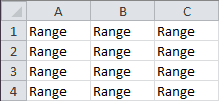Later, we’ll dedicate an entire lesson to the Dim Statement.

#### Value Property

Although it was optional, I used the Value Property in my Declaring a Range example, earlier. Let’s see another example. Say you have a value in one cell that you want to repeat in another range:

Open a worksheet and type a “3” in cell “A1.”

``````Sub RangeDemo()
Range("C1:D5") = Range("A1").Value
End Sub``````

Result: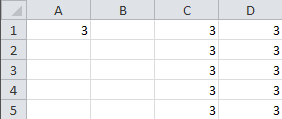#### Formula Property

``````Sub RangeDemo()
Range("C1:D5") = Range("A1").Value
Range("B5").Formula = "=Sum(C1:D5)"
MsgBox (Range("B5").Formula & vbNewLine & Range("B5").Value)
End Sub``````

Result: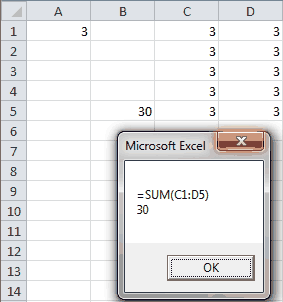You can see the Formula Property in the `MsgBox` returns a string that shows the formula, while the Value Property returns the output of the formula.

``````Sub RangeDemo()
End Sub``````

Result: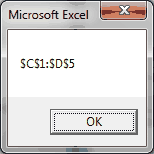In this example, the Address Property returns a string representing the location of the range. This is useful if working with Named Ranges or variable range dimensions.

#### Count Property

Want to know how big your range is? Or more useful, how many rows and how many columns your range is?

``````Sub RangeDemo()
Dim rng As Range
Set rng = Range("A1:C4")
rng.Value = "Range"
Range("A5") = rng.Count
Range("B5") = rng.Rows.Count
Range("C5") = rng.Columns.Count
End Sub``````

Result: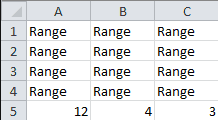The Range.Count Property returns the size of the complete range, 12. The Rows.Count Property returns the number of rows in the range, 4. The Columns.Count Property returns the number of columns in the range, 3.

If you’re looking for the last used cell in a range, I encourage you to read about the Range.End Property.

#### Copy and Paste

There are many ways to copy and paste in VBA, many of which start with the Copy Property.

##### Method 1: Range.Copy Method
``````Sub RangeDemo()
Range("A1:B3") = 7
Range("A1:B3").Copy Destination:=Range("a5")
End Sub``````

Result: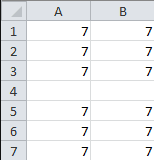##### Method 2: Worksheet.Paste Method
``````Sub RangeDemo()
Range("A1:B3").Copy
Range("A5").Select
ActiveSheet.Paste
End Sub``````

Result: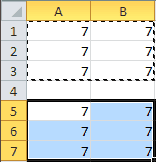##### Method 3: Range.PasteSpecial Method
``````Sub RangeDemo()
Range("A1:B3").Copy
Range("A5").PasteSpecial
End Sub``````

Result:Notice there’s no Range.Paste method.

#### ClearContents Method

If you want to clear the contents of a range, there’s a method for that.

``````Sub RangeDemo()
Range("A1:B3") = 7
Range("A5:B7") = Range("A1:B3").Value
Range("A1:B3").ClearContents
End Sub``````

Result: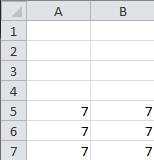This example takes a range from one location, moves it to another and clears the first range. The ClearContents Method erases the values in a range, while the Clear Method erases the values and the formatting.

#### PrintOut Method

Want to print a range?

``````Sub RangeDemo()
Range("A1:B3") = 7
Range("A1:B3").PrintOut
End Sub``````

The PrintOut Method will print your desired range to your default printer. Altenerately, the PrintPreview Method will pull up the print preview of what your range would look like if you chose to print it.

In this lesson you learned how to control your spreadsheet with the Range Object. You learned many Range Properties, including the Value, Formula, Address and Count Properties. You also learned many Range Methods, including the Copy, ClearContents and Printout Methods.

We didn’t touch much on the Select Method in this lesson. The Select Method is important enough that we’re dedicating the entire next lesson toward it. I hope to see you there.

If you really want to learn VBA, grab a copy of our Ultimate VBA Training Bundle before reading our next tutorial. We specifically created these cheat sheets to help you get the most out of our upcoming lessons. Together, the set has over 200 practical tips covering the 125 most important topics in Excel VBA. We also stuffed it with 140 helpful macro examples.